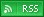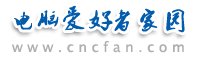电脑爱好者，提供IT资讯信息及各类编程知识文章介绍，欢迎大家来本站学习电脑知识。 最近更新 | 联系我们站内搜索：

# Asp编码优化技巧8则_提交运行效率

1.声明VBScript变量

2.对URL地址进行编码

HTTP Error 400
Due to malformed syntax, the request could not be understood by the server.
The client should not repeat the request without modifications.

<%
URL="xur.asp"
var2="&company=" & server.URLencode("xurstudio")
var3="&phone=" & server.URLencode("021-53854336-186")
response.redirect URL & "?" & var1 & var2 & var3
%>

3.清空对象

<% myDSN="DSN=xur;uid=xur;pwd=xur"
mySQL="select * from authors where AU_ID<100"
conntemp.open myDSN
set rstemp=conntemp.execute(mySQL)
if rstemp.eof then
response.write "数据库为空"
response.write mySQL
conntemp.close
set conntemp=nothing
response.end
end if%>
<%do until rstemp.eof %>
<%
rstemp.movenext
loop
rstemp.close
set rstemp=nothing
conntemp.close
set conntemp=nothing
%>

4.使用字符串建立SQL查询

<%mySQL= ""select * "
mySQL= mySQL & "from publishers"
mySQL= mySQL & "where state=’NY’"
response.write mySQL
set rstemp=conntemp.execute(mySQL)
rstemp.close
set rstemp=nothing
%>

5.使用case进行条件选择

<%
FOR i = 1 TO 1000
n = i
NEXT
%>
<%
numpart = RIGHT(num,1)
SELECT CASE numpart
CASE "1"
IF InStr(num,"11") THEN
num = num & "th"
ELSE
num = num & "st"
END IF
CASE "2"
IF InStr(num,"12") THEN
num = num & "th"
ELSE
num = num & "nd"
END IF
CASE "3"
IF InStr(num,"13") THEN
num = num & "th"
ELSE
num = num & "rd"
END IF
CASE "4"
num = num & "th"
CASE ELSE
num = num & "th"
END SELECT
END FUNCTION
%>

<%
connectme="DSN=xur;uid=xur;pwd=xur"
sqltemp="select * from publishers where name=’xur’"
response.write rstemp.recordcount & " records in<br>" & sqltemp
rstemp.close
set rstemp=nothing
%>

7.避免在使用global.asa文件中进行对象定义

<%SUB application_onstart
END SUB %>;

<%
mySQL="select * from publishers where state=’xur’
set rstemp=application("theconn").execute(mySQL)
%>

<%SUB session_onstart
END SUB %>

<%
mySQL="select * from publishers where state=’xur’
set session("rstemp")=conntemp.execute(mySQL)
%>

<!--#INCLUDE VIRTUAL="/define.asp" -->

8.安全防护
ASP提供了很好的代码保护机制，所有的ASP代码都在服务器端执行而只返回给客户端代码执行结果。即便这样，在老版本的IIS中还可以在文件名后面加::\$DATA来查看ASP的源代码，这已经属于Web Server安全范畴不在本文讨论范围内。下面提出两点简单的安全注意事项。

 Asp热门文章排行 网站赞助商